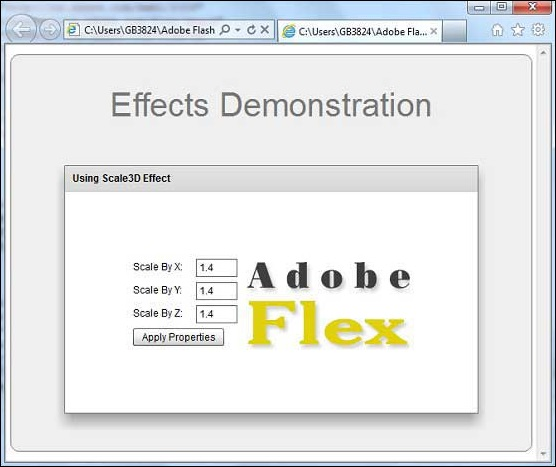# Flex - Scale3D Effect

## Introduction

The Scale3D class scales a target object in three dimensions around the transform center. A scale of 2.0 means the object is magnified by a factor of 2, and a scale of 0.5 means the object is reduced by a factor of 2.

## Class Declaration

Following is the declaration for spark.effects.Scale3D class −

```public class Scale3D
extends AnimateTransform3D
```

## Public Properties

Sr.No Property & Description
1

scaleXBy : Number

The factor by which to scale the object in the x direction.

2

scaleXFrom : Number

The starting scale factor in the x direction.

3

scaleXTo : Number

The ending scale factor in the x direction.

4

scaleYBy : Number

The factor by which to scale the object in the y direction.

5

scaleYFrom : Number

The starting scale factor in the y direction.

6

scaleYTo : Number

The ending scale factor in the y direction.

7

scaleZBy : Number

The factor by which to scale the object in the z direction.

8

scaleZFrom : Number

The starting scale factor in the z direction.

9

scaleZTo : Number

The ending scale factor in the z direction.

## Public Methods

Sr.No Method & Description
1

Scale3D(target:Object = null)

Constructor.

## Methods Inherited

This class inherits methods from the following classes −

• spark.effects.AnimateTransform3D
• spark.effects.AnimateTransform
• spark.effects.Animate
• mx.effects.Effect
• flash.events.EventDispatcher
• Object

## Flex Scale3D Effect Example

Let us follow the following steps to check usage of Scale3D Effect in a Flex application by creating a test application −

Step Description
1 Create a project with a name HelloWorld under a package com.tutorialspoint.client as explained in the Flex - Create Application chapter.
2 Modify HelloWorld.mxml as explained below. Keep rest of the files unchanged.
3 Compile and run the application to make sure business logic is working as per the requirements.

Following is the content of the modified mxml file src/com.tutorialspoint/HelloWorld.mxml.

```<?xml version = "1.0" encoding = "utf-8"?>
width = "100%" height = "100%" minWidth = "500" minHeight = "500">

<fx:Style source = "/com/tutorialspoint/client/Style.css" />
<fx:Script>
<![CDATA[
private function applyScaleProperties():void {
scaleEffect.play();
}
]]>
</fx:Script>

<fx:Declarations>
<s:Scale3D id = "scaleEffect" target = "{imageFlex}"
scaleXFrom = "1.0" scaleXTo = "{Number(scalingX.text)}"
scaleYFrom = "1.0" scaleYTo = "{Number(scalingY.text)}"
scaleZFrom = "1.0" scaleZTo = "{Number(scalingZ.text)}"
/>
</fx:Declarations>

<s:BorderContainer width = "630" height = "480" id = "mainContainer"
styleName = "container">
<s:VGroup width = "100%" height = "100%" gap = "50"
horizontalAlign = "center" verticalAlign = "middle">

<s:Label id = "lblHeader" text = "Effects Demonstration"
fontSize = "40" color = "0x777777" styleName = "heading" />
<s:Panel id = "scale3DPanel" title = "Using Scale3D Effect"
width = "500" height = "300">

<s:layout>
<s:HorizontalLayout  gap = "10" verticalAlign = "middle"
horizontalAlign = "center" />
</s:layout>

<s:VGroup top = "10" left = "15">
<s:HGroup verticalAlign = "middle">
<s:Label text = "Scale By X:" width = "70" />
<s:TextInput id = "scalingX" text = "1.4" width = "50" />
</s:HGroup>

<s:HGroup verticalAlign = "middle">
<s:Label text = "Scale By Y:" width = "70" />
<s:TextInput id = "scalingY" text = "1.4" width = "50" />
</s:HGroup>

<s:HGroup verticalAlign = "middle">
<s:Label text = "Scale By Z:" width = "70" />
<s:TextInput id = "scalingZ" text = "1.4" width = "50" />
</s:HGroup>

<s:Button label = "Apply Properties" click = "applyScaleProperties()" />
</s:VGroup>

<s:Image id = "imageFlex"
source = "http://www.tutorialspoint.com/images/flex-mini.png" />
</s:Panel>
</s:VGroup>
</s:BorderContainer>
</s:Application>
```

Once you are ready with all the changes done, let us compile and run the application in normal mode as we did in Flex - Create Application chapter. If everything is fine with your application, it will produce the following result: [ Try it online ]flex_visual_effects.htm